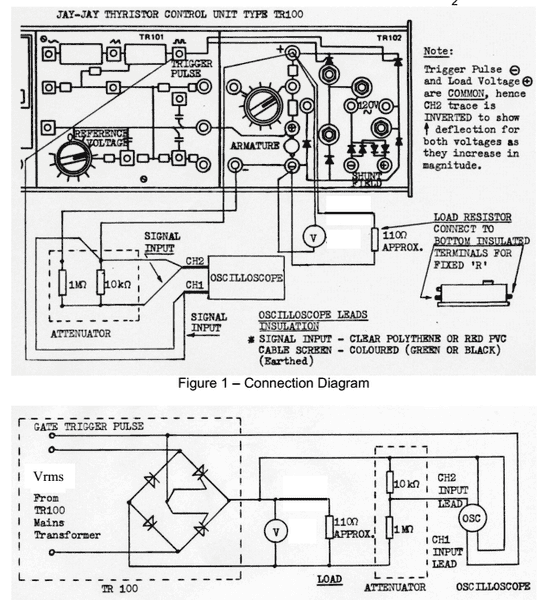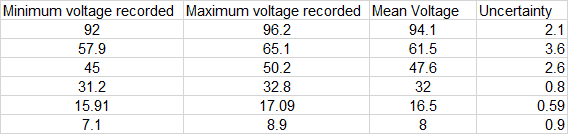# How to quantify relatively simple experimental errors

• urb-nurd

## Homework Statement

For my coursework task, i am to quantify and discuss errors associated with an experimental procedure.
This involved a thyristor control unit which allowed the firing angle of two thyristors (in a half controlled bridge rectifier) to be varied with a rotary dial or pot/rheo.The image above shows the hardware and the connection diagram supplied.
The output of the bridge was measured with a digital voltmeter.
This output voltage was recorded at various firing angles where the angle is determined on an oscilloscope trace.
Theoretical calculations were also carried out from the formulae : Vout = (√2 * Vrms) / π)*(1+cos(α))
Where Vrms is the measured AC input value and alpha corresponds to the firing angle.
These theoretical values are to be plotted over a range of 0-180 degrees on a graph along with the measured values.
I am tasked to quantify the error associated with the procedure and equipment so as to calculate an overall error.
The total error is to be represented by error bars on the graph showing theoretical results and measured results.

## Homework Equations

My question, is what errors can be quantified here?
I know there is an error associated the volt meter - ± 1/2 of the least significant figure.
This causes an inherent error in my theoretical calculation i believe.

The firing angle of the half controlled bridge is determined on the oscilloscope, introducing a measurement error of ± 1/2 of the lowest resolution on the scale. For my set up, this is ± 4.5 degrees.
The volt meter output also fluctuated quite a-lot when taking readings causing my recorded voltages to be no more than an approximation of the average value, also due to time constraints of the lab - i have been limited to a single set of measurements
I believe these to be to be the dominant quantifiable errors - Voltage reading and angle reading that is.

## The Attempt at a Solution

I think i have quantified the errors appropriately:
Voltmeter error = ±0.5V (half the least significant figure of the output)
Firing Angle reading error = ± 4.5 degrees (given that my scope is set to 9 degree per sub unit and 25 per unit on the scale).

If these two errors are agreed to be the dominant and relevant errors associated with the circuit above, how can i display these correctly as error bars?

I know my theoretical calculations have a level of error based on the error of measured voltage value that the formula utilizes. Is it correct to display this as vertical error bars on my theoretical line? (the percentage error is 0.4% in the theoretical calculation)

The measured values also have this voltmeter error along with an error in reading the corresponding firing angle.
How can i combine these two to produce a total error?
Or do i display these two errors on their relevant axis oriented error bars? given that the error in angle reading can be displayed as a horizontal error bar on the measured values in the graph, and the voltage reading error can be displayed as vertical error bars on the measured values in the graph.

Any information or advice that may help me understand where to go from here is greatly appreciated!
I do apologize for any inadequacies or errors in my formatting.
Thanks !
[/B]

I have attached a picture of my results graphed to help visualise.

One thing to be leery about is the behavior of any given voltmeter when using it to read non pure DC or non sinusoidal voltages. The old analog meter movements were pretty good at measuring average values due to the inertia of the coil movement. Modern digital voltmeters are at the whim of manufacturer's choice on how to sample and process the readings. The better ones have "true RMS" processing built in. You might want to see if you can dig up the manufacturer's specs on the meter you used for the lab to see what it does with these things. Who knows, they might have a correction method for certain situations.

The measured values also have this voltmeter error along with an error in reading the corresponding firing angle.
How can i combine these two to produce a total error?
Or do i display these two errors on their relevant axis oriented error bars? given that the error in angle reading can be displayed as a horizontal error bar on the measured values in the graph, and the voltage reading error can be displayed as vertical error bars on the measured values in the graph.

That's a good question!

If there is any "industrial standard" for displaying (x,y) data when there are possible measurement errors in both x and y, you should be able to find examples of it. Likewise, if the method your course expects you to use is peculiar to the course, you should look for examples presented in the course.

Lacking specific directions, my personal choice would be to indicated them both vertically and horizontally about the (x,y) data by a box.

This webpage aimed toward biologists indicates (in section 13) that the "Excel" program has an option for x error bars. I don't use Excel myself, so I don't know what that option does. http://www.biostathandbook.com/graph.html

Thanks for the input.

One thing to be leery about is the behavior of any given voltmeter when using it to read non pure DC or non sinusoidal voltages. The old analog meter movements were pretty good at measuring average values due to the inertia of the coil movement. Modern digital voltmeters are at the whim of manufacturer's choice on how to sample and process the readings. The better ones have "true RMS" processing built in. You might want to see if you can dig up the manufacturer's specs on the meter you used for the lab to see what it does with these things. Who knows, they might have a correction method for certain situations.
The voltmeter used is of such age that I couldn't find a single scrap of a datasheet when i did some digging through google.

That's a good question!

If there is any "industrial standard" for displaying (x,y) data when there are possible measurement errors in both x and y, you should be able to find examples of it. Likewise, if the method your course expects you to use is peculiar to the course, you should look for examples presented in the course.

Lacking specific directions, my personal choice would be to indicated them both vertically and horizontally about the (x,y) data by a box.

This webpage aimed toward biologists indicates (in section 13) that the "Excel" program has an option for x error bars. I don't use Excel myself, so I don't know what that option does. http://www.biostathandbook.com/graph.html

This particular module didn't have any error analysis in the theory, which is why is it slightly strange that errors appear to be the main focus of the assignment.
That means there is no expected method prescribed, though i am sure my lecturer knows exactly what he is expecting - they just likes to watch us squirm as we waste critical time trying to gauge this expectation with no assistance.

Thanks for the link, i will refer to that if i get stuck with excel.

I actually have error bars on both results above, the theoretical error bars correspond to the error in calculation stemming from a 0.5V error in the measured voltage.

Again, thanks for the input all!

Ok i have narrowed down my largest source of error to the random error in the voltmeter readings.As the table shows above, i recorded a maximum and a minimum that the reading was seen to repetitively fluctuate between. From this i can calculate the average voltage and its associated uncertainty.
What is the correct method for determining the overall absolute error of this data set?
Thanks again!